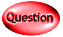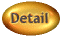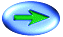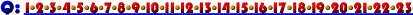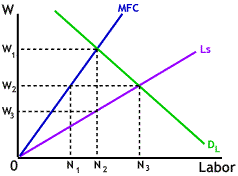12. If the firm whose data are depicted to the right acts as a profit maximizer it will choose which of the following wage-labor combinations?
1. Wage = W2 and Labor = N3
2. Wage = W1 and Labor = N2
3. Wage = W3 and Labor = N3
4. Wage = W3 and Labor = N2
5. Wage = W2 and Labor = N1